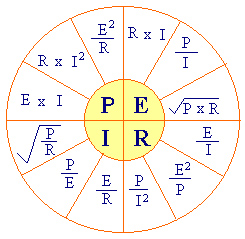Chapman Electric Supply, Inc. is now  part of Eckart Supply!  learn more>>

# Electrical Calculation Formulas

At Chapman Electric Supply, we’re committed to helping you get your job done right. Below you will find various charts to aid you in calculating your needs for a wide array of electrical applications. They are useful for both professional and hobby electricians. You can also find more articles in our resources section.

If you still have questions, contact us today!  We’re ready to help you tackle any problem. We have the know-how and many years of quality service and supply behind us.

## AC/DC Formulas

Use these formulas to calculate amps, kilowatts, kilovolts, and horsepower for different voltages and phases

 To Find Direct Current AC / 1phase 115v or 120v AC / 1phase 208,230, or 240v AC 3 phase All Voltages Amps when Horsepower is Known HP x 746 E x Eff HP x 746 E x Eff X PF HP x 746 E x Eff x PF HP x 746 1.73 x E x Eff x PF Amps when Kilowatts is known kW x 1000 E kW x 1000 E x PF kW x 1000 E x PF kW x 1000 1.73 x E x PF Amps when kVA is known kVA x 1000 E kVA x 1000 E kVA x 1000 1.73 x E Kilowatts I x E 1000 I x E x PF 1000 I x E x PF 1000 I x E x 1.73 PF 1000 Kilovolt-Amps I x E 1000 I x E 1000 I x E x 1.73 1000 Horsepower (output) I x E x Eff 746 I x E x Eff x PF 746 I x E x Eff x PF 746 I x E x Eff x 1.73 x PF 746

 Key: E = Voltage I = Amps W = Watts PF = Power Factor Eff = Efficiency HP = Horsepower

## Ohm's Law

Ohm’s Law consists of the mathematical relationship between Voltage, Current and Resistance. Ohm's Law states that for an electrical circuit, the current is directly proportional to the voltage and inversely proportional to the resistance.

### Ohm’s Law Pie ChartP = watts I = amps R = ohms E = Volts

## AC Efficiency & Power Factor Formulas

### AC Efficiency

 To Find Single Phase Three Phase Efficiency 746 x HP E x I x PF 746 x HP E x I x PF x 1.732 Power Factor Input Watts V x A Input Watts E x I x 1.732

### Power – DC Circuits

 Watts = E xI Amps = W / E

 Key: E = Voltage I = Amps W = Watts PF = Power Factor Eff = Efficiency HP = Horsepower

## Voltage Drop Formulas Chart

 Single Phase (2 or 3 wire) VD = 2 x K x I x L CM K = ohms per mil foot  (Copper = 12.9 at 75°) (Alum = 21.2 at 75°) L = Length of conductor in feet I  = Current in conductor (amperes) CM = Circular mil area of conductor CM= 2K x L x I VD Three Phase VD= 1.73 x K x I x L CM CM= 1.73 x K x L x I VD

Note: K value changes with temperature.

cs